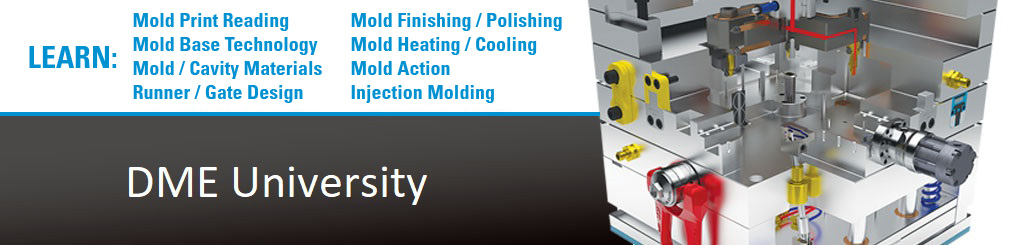# Determining Cooling Requirements

Many mold designers determine cooling requirements by previous experience. Some mold designers determine cooling requirements by using a zone cooling method. A given coolant hole size will zone cool a specific diameter around the coolant hole. Mold cooling requirements can be calculated and there are computer software programs available to simplify the time and work involved using calculations. The following content will give you a better appreciation of what is involved in calculations:

• Equation A is for calculating the heat content of plastic material entering the mold.
• Equation B is used to calculate convection, or the ability of a medium to carry heat from one place to another.
• Equation C is for the calculation of conduction or transferring heat from two bodies in contact.
• Equation D is the Reynolds number equation. It is preferred to have a turbulent (agitated or distributed) coolant flow through the cooling channels to gain better heat transfer. The Reynolds number is a measure of turbulent flow.

Previous

Next

Page 7 of 24